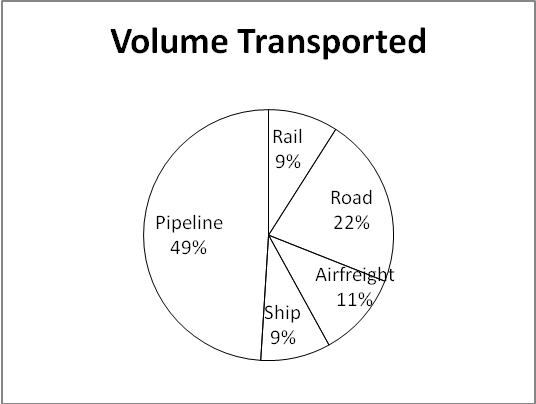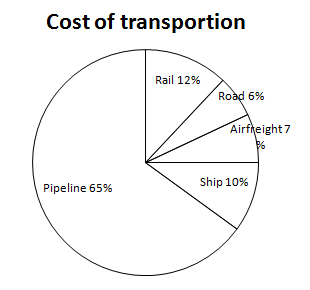# Crack IBPS PO : data interpretation : Aptitude day 56

D.1-5) Chart 1 shows the distribution of twelve million tonnes of courier transport through different modes over a specific period of time. Chart 2 shows the distribution of the cost of transporting this courier. The total cost was Rs.30 million.Q.1) What is the cost of transporting courier by rail per ton(in Rs)?

a) 2.5

b) 3.33

c) 6.4

d) 8

e) None of these

(b)

We get 3.6/1.08=3.33

Q.2) If the cost per ton of transport by ship, airfreight and road are represented by P, Q and R respectively, which of the following is true?

a) R > Q > P

b) P > R > Q

c) P > Q > R

d) R > P > Q

e) None of these

(c)

Calculate the cost by ship, air and road.
P = 3/1.08= 2.77

Q = 2.10/1.32= 1.59

R = 1.80/2.64= 0.68

hence P > Q > R

Q.3) Which is the most effective way of transportation?

b) Ship

c) Pipeline

d) can’t be determined

e) None of these

(d)

cannot be determined as  we do not know on what criteria

Q.4) If the pipeline cost increases by 30% , by what percentage would revenue have to be increased so as to have same amount of profit?

a) 11.5

b) 12.8

c) 13.75

d) 14.6

e) None of these

(d)

Pipeline cost is 65% of total cost =Rs.19.5 Million

If this rises by 30 %, it rises by 5.85 Million Cost of transportion for pipeline=19.5/5.8=3.36

Now Total cost=19.5+5.85=25.35

25.35/x=3.36

x=3.36×25.35=7.54

The percentage increase=(7.54-5.8)/12×100=14.54%

Revenue would have to rise by the same amount, so 100 = 14.625%

Q.5) If for some reason ship stop sailing, by what percentage the airfreight have to go up to reach the previous level of volume transported (approximately)?

a) 75%

b) 81%

c) 85%

d) 90%

e) None of these

(b)

Air freight is 11% .it would have to increase to 20% i.e. increase by  9/11×100=81.81%

D.6-10) What approximate value will come in place of question mark (?) in the given question? (You are not expected to calculate the exact value).

Q.6) 105.27 % of 1200.11 + 11.80% of 23600.85 = 21.99% of (?) + 1420.99

a) 5000

b) 2400

c) 3100

d) 12140

e) 9600

(d)

105.27% of 1200.11+ 11.08% of 23600.85 = 21.99% of ? + 1420.99

= 105% of 1200 + 12% of 23600 = 22% of ? + 1421

= 1260 + 2832 = 0.22 ×? + 1421

= 0.22 ×?= 2671

= ? (2671 ÷ 0.22) = 12140.90 ≈12140

Q.7) 0.98% of 7824 + 4842 ÷ 119.46 – (?) = 78

a) 30

b) 60

c) 40

d) 50

e) 70

(c)

0.98% of 7824 + 4842 ÷ 119.46 – ? = 78

1% of 7824 + 4842 ÷ 119 – 78 =?

= ? = 78.24 + 40.68 – 78 = 40.92 ≈ 40

Q.8) (42.1992 –18.1642 )(2 )-(?) = 168.62 – 138.99

a) 4004

b) 1200

c) 172

d) 867

e) 546

(e)

(42.1992 – 18.1642 )2– ? = 168.62 – 138.99

=>(42 – 18)2 – ? = 169 – 139

(24)2-?=30

?=546

Q.9) (?)3+ √8– 340 = (√8×√8)(1/2)+ (9)(1/2)

a) 7

b) 19

c) 18

d) 9

e) None of these

(a)

(?)3 + √8 – 340 = (√8×√8)(1/2) + (9)(1/2)

(?)3 + √8 – 340 = √8 + 3

(?)3 = √8 + 3 – √8 + 340

(?)3 = 343

? = ∛343

? = 7

Q.10) (15 × 0.40)4÷ (1080 ÷ 30)4 × (27 × 8)4 = (3 × 2)?×65

a) 8

b) 3

c) 12

d) 16

e) None of these

(b)

= (15 × 0.40)4÷(1080 ÷ 30)4×(27 × 8)4=(3 × 2)?×65

=  (6)4÷(36)4×(216)4=(6)?×(6)5

=(6)?=(6)8/(6)5

? + 5 = 8

?=3